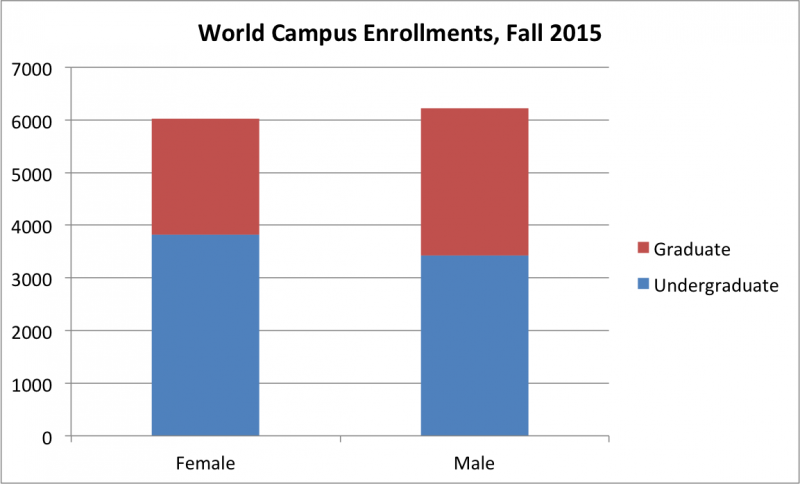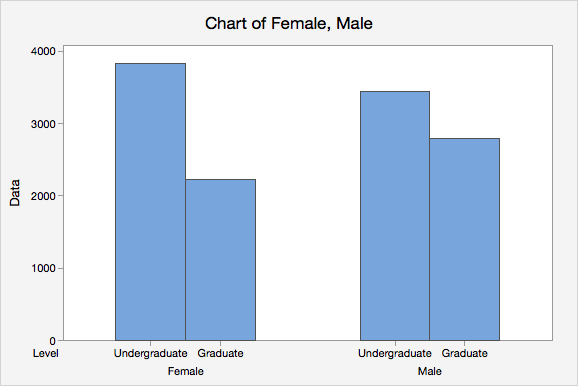# 2.1.2 - Two Categorical Variables

Data concerning two categorical variables may be communicated using a two-way table, also known as a contingency table. Data concerning two categorical variables can visualized using a segmented bar chart or a clustered bar chart. A clustered bar chart is also known as a side-by-side bar chart.

Two-Way Table
A display of counts for two categorical variables in which the rows represent one variable and the columns represent a second variable. Also known as a contingency table.

## Example: World Campus Enrollments by Sex Section

We will use the two-way table below of Penn State World Campus enrollments by biological sex and level to walk through a few examples of how to read a two-way table. These data are from the Penn State Factbook and from the Fall of 2015.

Female Male Total
Total 6027 6215 12242

What proportion of the population of World Campus students is undergraduate?

$$p=\frac{7242}{12242}=0.596$$

#### Proportion of Females who are Undergraduates

What proportion of females in this population are undergraduate students?

$$p=\frac{3814}{6027}=0.633$$

Later in this lesson, you will learn that this is known as a conditional probability.

#### Proportion of Undergraduates who are Female

What proportion of undergraduate students in this population are female?

$$p=\frac{3814}{7242}=0.527$$

Segmented Bar Chart

Also known as a stacked bar chart, one categorical variable is represented on the x-axis while the second categorical variable is denoted within the bars. Minitab Express will not construct a stacked bar chart, but other softwares will. The segmented bar chart below was constructed using Excel.Clustered Bar Chart

Each bar represents one combination of the two categorical variables (i.e., one cell in a contingency table). This is also known as a side-by-side bar chart.